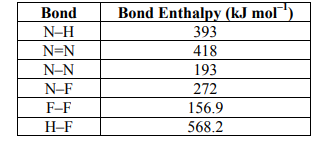# Problem: Calculate the magnitude of the enthalpy change, and determine if the reaction is exothermic or endothermic for the following reaction: NH3 + 3F2 ⟶ NF3 + 3HFA. 870.9 kJ mol–1 , exothermic B. 870.9 kJ mol–1 , endothermic C. 1112 kJ mol–1 , exothermic D. 1112 kJ mol–1 , endothermic E. 290.3 kJ mol–1 , endothermic

###### FREE Expert Solution

We are asked to calculate the magnitude of the enthalpy change, and determine if the reaction is exothermic or endothermic for reaction.

To calculate the ΔH°rxn using bond energies, we’re going to use the following equation:

Let’s first figure out what kind of bonds and how many moles are present in each reactants and products because the given bond energies are per mole.

Balanced Reaction NH3 + 3F2 ⟶ NF3 + 3HF

*always make sure that the given reaction is balanced

Draw the structure of each compound.

Reactants:

NH3:

97% (156 ratings)###### Problem Details

Calculate the magnitude of the enthalpy change, and determine if the reaction is exothermic or endothermic for the following reaction: NH3 + 3F2 ⟶ NF3 + 3HFA. 870.9 kJ mol–1 , exothermic

B. 870.9 kJ mol–1 , endothermic

C. 1112 kJ mol–1 , exothermic

D. 1112 kJ mol–1 , endothermic

E. 290.3 kJ mol–1 , endothermic